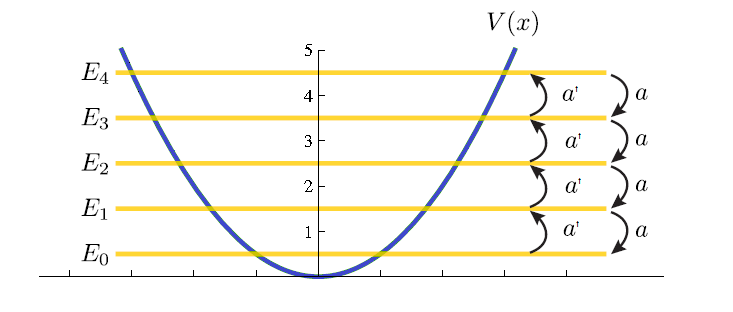Ladder Operators are operators that increase or decrease eigenvalue of another operator. There are two types; raising operators and lowering operators. In quantum mechanics the raising operator is called the creation operator because it adds a quantum in the eigenvalue and the annihilation operators removes a quantum from the eigenvalue. For example, in quantum harmonic oscillator, creation operators adds a quantum of energy to the system and annihilation operators removes a quantum of energy from the system. While in potential system quantum field theories, creation operators add a particle to the system and annihilation operators remove a particle from the system.

Ladder operators increase or decrease eginvaules by a quantum. An example of ladder operators are the a type of angular momentum operators.

Creation operator:

${L_+ Y_{\ell m}}={\hbar}{\sqrt{\ell(\ell+1)-m(m+1)}}{Y_{\ell(m+1)}}$

Annihilation operator:

${L_- Y_{\ell m}}={\hbar}{\sqrt{\ell(\ell+1)-m(m-1)}} {Y_{\ell(m-1)}}$

The creation operators increases the value of m and the annihilation operators decreases the value of m, both without affecting the value of l.

Below is a graphical representation of what ladder operators do when related to energy eigenvalue of the quantum harmonic oscillator. The Creation operators at increases the energy value by a quantum and the annihilation operator decreases the the energy value by a quantum.In angular momentum, the ladder operators are J+ and J-.

$J_-=J_x-iJ_y$

$J_+=J_x+iJ_y$

Then with the operator Jz

$J_z J_\pm|j m \rangle =(J_\pm J_z +[J_z,J_\pm]) |jm \rangle$

$J_z J_\pm|j m \rangle =(J_\pm J_z \pm \hbar J_\pm)|jm \rangle$

$J_z J_\pm|j m \rangle = \hbar (m \pm 1) J_\pm |jm \rangle$

therefore

$J_z |j m \pm 1 \rangle = \hbar (m \pm 1)|jm \rangle$

so we can see that

Creation Operator:

$J_+|j m \rangle =\alpha|jm+1 \rangle$

Annihilation Operator:

$J_-|j m \rangle =\beta|m-1 \rangle$

Where

$\alpha=\hbar \sqrt{(j-m)(j+m+1)}|jm+1 \rangle$

$\beta=\hbar \sqrt{(j+m)(j-m+1)}|jm-1 \rangle$

This shows why ladder operators are called creation and annihilation operators. The operators relate one eigenvalue to the next one by lower or rising the a quantum number.

## Quantum Harmonic Oscillator Ladder Operator

Another example of ladder operators is for the quantum harmonic oscillator. The ladder operators for quantum harmonic oscillator rise or lower the energy of the system by a quantam.

To see where the operators come from, we start with the Schrödinger equation:

$\left(-\frac{\hbar^2}{2m}\frac{d^2}{dx^2}+\frac{1}{2}m\omega^2x^2\right)\psi(x)=E\psi(x)$

Setting x as

$x=\sqrt{\frac{\hbar}{m\omega}}q$

The Schrödinger equation is now

$\frac{\hbar\omega}{2}\left(-\frac{d^2}{dq^2}+q^2\right) \psi(q)=E\psi(q)$

$\hat H=-\frac{d^2}{dq^2}+q^2$

$\hat H=\left(-\frac{d}{dq}+q\right)\left(\frac{d}{dq}+q\right)+1$

So now the Schrödinger equation becomes

$\hbar\omega \left[\frac{1}{\sqrt2} \left(-\frac{d}{dq}+q\right) \frac{1}{\sqrt2} \left(\frac{d}{dq}+q\right) + \frac{1}{2}\right] \psi(q)=E\psi(q)$

Creation Operator:

$a^t=\frac{1}{\sqrt 2} \left(-\frac{d}{dq}+q \right )$

Annihilation Operator:

$a=\frac{1}{\sqrt 2} \left (\frac{d}{dq}+q \right )$

From this we can get our equation (we won't do it here) for the energy of an our eigenvaules.

$E_n=(n+\frac{1}{2})\hbar\omega$

## Conclusion

There are two kinds of ladder operators, creation and annihilation operators. Like the word ladder suggests, these operators move eigenvalues up or down. They are used in angular momentum to rise or lower quantum numbers and quantum harmonic oscillators to move between energy levels.

## Contributors

• Kevin Zhang (Fall 2016)